# EV design – battery calculation

The high voltage battery it is one of the most important component of a battery electric vehicle (BEV). The battery parameters have a significant influence on other components and attributes of the vehicle, like:

• maximum traction motor torque
• maximum regeneration brake torque
• vehicle range
• vehicle total weight
• vehicle price

Pretty much all major aspects of a pure electric vehicle (EV) depend on the parameters of the high voltage battery.

For our electric vehicle battery design we are going to start from 4 core input parameters:

• chemistry
• voltage
• average energy consumption of the vehicle on a driving cycle
• vehicle range

A battery consists of one or more electrochemical cells (battery cells) which are converting chemical energy into electrical energy (during discharging) and electrical energy into chemical energy (during charging). The type of elements contained within a battery and the chemical reactions during discharging-charging events define the chemistry of a battery.

A battery cell consists of five major components: electrodes – anode and cathode, separators, terminals, electrolyte and a case or enclosure. For automotive applications there are different types of cells used :

Individual battery cells are grouped together into a single mechanical and electrical unit called a battery module. The modules are electrically connected to form a battery pack.

There are several types of batteries (chemistry) used in hybrid and electric vehicle propulsion systems but we are going to consider only Lithium-ion cells. The main reason is that Li-ion batteries have higher specific energy [Wh/kg] and specific power [W/kg] compared with other types .

The voltage level of the battery determines the maximum electrical power which can be delivered continuously. Power P [W] is the product between voltage U [V] and current I [A]:

$P = U \cdot I \tag{1}$

The higher the current, the bigger the diameter of the high voltage wires and the higher the thermal losses. For this reason, the current should be limited to a maximum and the nominal power obtained by having a higher voltage. For our application we are going to consider a nominal voltage of 400 V.

In the article EV design – energy consumption we have calculated the average energy consumption for propulsion Ep as being 137.8 Wh/km on WLTC drive cycle. On top of the energy needed for propulsion, the high voltage battery must supply the energy for the vehicle’s auxiliary devices Eaux [Wh/km], like: 12 V electrical system, heating, cooling, etc. Also, we have to consider the efficiency of the powertrain ηp [-] during the conversion from electrical energy to mechanical energy.

$E_{avg} = \left ( E_{p} + E_{aux} \right ) \cdot \left ( 2 – \eta_{p} \right ) \tag{2}$

For the auxiliary devices energy consumption we are going to use data from , which contains the typical power requirements of some common vehicle electrical components (auxiliary loads). The prolonged electrical loads (headlights, multimedia, etc.) and intermittent loads (heater, brake lights, wipers, etc.) use on average 430 W of electrical power. The duration of the WLTC cycle is 1800 s (0.5 h), which gives an energy of 215 Wh for the auxiliary loads. If we divide it to the length of the WLTC driving cycle (23.266 km), we get an average energy consumption for the auxiliary loads Eaux of 9.241 Wh/km.

Even if Wh/km is not really energy but factorised energy, since it’s dived per unit of distance (km), for simplicity, we are going to refer to it as average energy.

The direct current (DC) supplied by the battery is converted into alternated current (AC) by the inverter. This conversion is taking place with an associated loss. Also, the electric motor and driveline have some losses which we need to consider. For this exercise we are going to use an average efficiency ηp of 0.9 from the battery to the wheel.

Replacing the values in (2) gives the average energy consumption:

$E_{avg} = \left ( 137.8 + 9.241 \right ) \cdot 1.1 = 161.7451 \text{ Wh/km}$

The battery pack will be designed for an average energy consumption of 161.7451 Wh/km.

### Battery pack architectures

All high voltage battery packs are made up from battery cells arranged in strings and modules. A battery cell can be regarded as the smallest division of the voltage.

Individual battery cells may be grouped in parallel and / or series as modules. Further, battery modules can be connected in parallel and / or series to create a battery pack. Depending on the battery parameters, there may be several levels of modularity.

The total battery pack voltage is determined by the number of cells in series. For example, the total (string) voltage of 6 cells connected in series will be the sum of their individual voltage.

In order to increase the current capability the battery capacity, more strings have to be connected in parallel. For example, 3 strings connected in parallel will triple the capacity and current capability of the battery pack.

The high voltage battery pack of Mitsubishi i-MiEV consists of 22 modules made up from 88 cells connected in series. Each module contains 4 prismatic cells. The voltage of each cell is 3.7 V and the total voltage of the battery pack 330 V.

Another example is the high voltage battery pack of Tesla Model S, which has:

• 74 cells in a parallel group
• 6 groups in series for a module
• 16 modules in series
• 7104 cells in total

### Battery pack calculation

In order to chose what battery cells our pack will have, we’ll analyse several battery cells models available on the market. For this example we are going to focus only on Lithium-ion cells. The input parameters of the battery cells are summarised in table below.

Note: Since battery cells manufacturers come up with newer models continuously, it might be the case that the data used in this example is obsolete. This is less important since the aim or the article is to explain how the calculation is done. The same method can be applied for any other battery cells.

 Manufacturer Panasonic A123-Systems Molicel A123-Systems Toshiba Kokam Type cylindrical cylindrical cylindrical pouch pouch pouch Model NCR18650B ANR26650m1-B ICR-18650K 20Ah 20Ah SLPB7570270 Source       Length [m] 0.0653 0.065 0.0652 0 0 0 Diameter [m] 0.0185 0.026 0.0186 0 0 0 Height [m] 0 0 0 0.227 0.103 0.272 Width [m] 0 0 0 0.16 0.115 0.082 Thickness [m] 0 0 0 0.00725 0.022 0.0077 Mass [kg] 0.0485 0.076 0.05 0.496 0.51 0.317 Capacity [Ah] 3.2 2.5 2.6 19.5 20 15.6 Voltage [V] 3.6 3.3 3.7 3.3 2.3 3.6 C-rate (cont.) 1 10 1 1 1 2 C-rate (peak) 1 24 2 10 1 3

Based on the cell parameters provided by the manufacturers, we can calculate the energy content, volume, gravimetric density and volumetric density for each cell.

The volume of each cell is calculated as:

• cylindrical cells, Vcc [m3]
$V_{cc} = \frac{\pi D_{bc}^2}{4} \cdot L_{bc} \tag{1}$

where:
Dbc [m] – battery cell diameter
Lbc [m] – battery cell length

• pouch cells, Vpc [m3]
$V_{pc} = H_{bc} \cdot W_{bc} \cdot T_{bc} \tag{2}$

where:
Hbc [m] – battery cell height
Wbc [m] – battery cell width
Tbc [m] – battery cell thickness

The battery cell energy Ebc [Wh] is calculated as:

$E_{bc} = C_{bc} \cdot U_{bc} \tag{3}$

where:
Cbc [Ah] – battery cell capacity
Ubc [V] – battery cell voltage

The battery cell energy density is calculated as:

• volumetric energy density, uV [Wh/m3]
$u_{V} = \frac{E_{bc}}{V_{cc(pc)}} \tag{4}$
• gravimetric energy density, uG [Wh/kg]
$u_{G} =\frac{E_{bc}}{m_{bc}} \tag{5}$

where:
mbc [kg] – battery cell mass

The energy density for each cells are summarised in the table below.

 Manufacturer Panasonic A123-Systems Molicel A123-Systems Toshiba Kokam Type cylindrical cylindrical cylindrical pouch pouch pouch Model NCR18650B ANR26650m1-B ICR-18650K 20Ah 20Ah SLPB7570270 Energy [Wh] 11.52 8.25 9.62 64.35 46 56.16 Volume [l] 0.017553 0.034510 0.017716 0.263320 0.260590 0.171741 Energy density gravimetric [Wh/kg] 237.53 108.55 192.40 129.74 90.20 177.16 Energy density volumetric [Wh/l] 656.31 239.06 543.01 244.38 176.52 327

In order to have a better overview of the cells parameters and to compare them easier, the main parameters are displayed as bar graphs in the images below.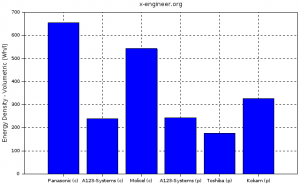Image: Battery cell volumetric energy density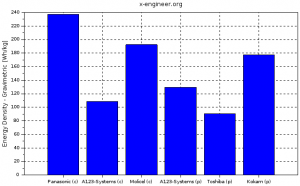Image: Battery cell gravimetric energy density

With the above cell parameters and the core requirements for the battery (nominal voltage, average energy consumption and vehicle range), we calculate the main parameters of the high voltage battery.

The required battery pack total energy Ebp [Wh] is calculated as the product between the average energy consumption Eavg [Wh/km] and vehicle range Dv [km]. For this example we’ll design the high voltage battery pack for a vehicle range of 250 km.

$E_{bp} = E_{avg} \cdot D_{v} = 161.7451 \cdot 250 = 40436.275 \text{ Wh} = 40.44 \text{ kWh} \tag{6}$

The following calculations are going to be performed for each cell type. For this example we are going to consider that the battery pack is made up only from several strings connected in parallel.

The number of battery cells connected in series Ncs [-] in a string is calculated by dividing the nominal battery pack voltage Ubp [V] to the voltage of each battery cell Ubc [V]. The number of strings must be an integer. Therefore, the result of the calculation is rounded to the higher integer.

$N_{cs} = \frac{U_{bp}}{U_{bc}} \tag{7}$

The energy content of a string Ebs [Wh] is equal with the product between the number of battery cells connected in series Ncs [-] and the energy of a battery cell Ebc [Wh].

$E_{bs} = N_{cs} \cdot E_{bc} \tag{8}$

The total number of strings of the battery pack Nsb [-] is calculated by dividing the battery pack total energy Ebp [Wh] to the energy content of a string Ebs [Wh]. The number of strings must be an integer. Therefore, the result of the calculation is rounded to the higher integer.

$N_{sb} = \frac{E_{bp}}{E_{bs}} \tag{9}$

We can now recalculate the battery pack total energy Ebp [Wh] as the product between number of strings Nsb [-] and the energy content of each string Ebs [Wh].

$E_{bp} = N_{sb} \cdot E_{bs} \tag{10}$

The battery pack capacity Cbp [Ah] is calculated as the product between the number of strings Nsb [-] and the capacity of the battery cell Cbc [Ah].

$C_{bp} = N_{sb} \cdot C_{bc} \tag{11}$

The total number of cells of the battery pack Ncb [-] is calculated as the product between the number of strings Nsb [-] and the number of cells in a string Ncs [-].

$N_{cb} = N_{sb} \cdot N_{cs} \tag{12}$

The size and mass of the high voltage battery are very important parameter to consider when designing a battery electric vehicle (BEV). In this example we are going to caclulate the volume of the battery pack considering only the battery cells. In reality there are other factors to consider, like: electronic circuits, cooling circuit, battery casing, wiring, etc.

The battery pack mass (cells only) mbp [kg] is the product between the total number of cells Ncb [-] and the mass of each battery cell mbc [kg].

$m_{bp} = N_{cb} \cdot m_{bc} \tag{13}$

The volume of the battery pack (cells only) Vbp [m3] is the product between the total number of cells Ncb [-] and the mass of each battery cell Vcc(pc) [m3]. This volume is only used to estimate the final volume of the battery pack, since it does not take into account the auxiliary components/systems of the battery.

$V_{bp} = N_{cb} \cdot V_{cc(pc)} \tag{14}$

The volume can be also calculated function of number of strings and number of cells in a string. This calculation method is more appropriate for cylindrical cell, since the volume occupied by a cylindrical cell must take into account the air gap between cells.

The string peak current Ispc [A] is the product between the peak C-rate of the battery cell C-ratebcp [h-1] and the battery cell capacity Cbc [Ah].

$I_{spc} = \text{C-rate}_{bcp} \cdot C_{bc} \tag{15}$

The battery pack peak current Ibpp [A] is the product between the string peak current Ispc [A] and the number of strings of the battery pack Nsb [-].

$I_{bpp} = I_{spc} \cdot N_{sb} \tag{16}$

The battery pack peak power Pbpp [W] is the product between battery pack peak current Ibpp [A] and the battery pack voltage Ubp [V].

$P_{bpp} = I_{bpp} \cdot U_{bp} \tag{17}$

The string continuous current Iscc [A] is the product between the continuous C-rate of the battery cell C-ratebcc [h-1] and the battery cell capacity Cbc [Ah].

$I_{scc} = \text{C-rate}_{bcc} \cdot C_{bc} \tag{18}$

The battery pack continuous current Ibpc [A] is the product between the string continuous current Iscc [A] and the number of strings of the battery pack Nsb [-].

$I_{bpc} = I_{scc} \cdot N_{sb} \tag{19}$

The battery pack continuous power Pbpc [W] is the product between battery pack continuous current Ibpc [A] and the battery pack voltage Ubp [V].

$P_{bpc} = I_{bpc} \cdot U_{bp} \tag{20}$

The results of the equations (7) to (20) are summarised in the table below.

 Manufacturer Panasonic A123-Systems Molicel A123-Systems Toshiba Kokam # of cells in string [-] 112 122 109 122 174 112 String energy [Wh] 1290 1007 1049 7851 8004 6290 # of strings [-] 32 41 39 6 6 7 BP energy [kWh] 41.29 41.27 40.89 47.10 48.02 44.03 BP capacity [Ah] 102.4 102.5 101.4 117 120 109.2 # total cells [-] 3584 5002 4251 732 1044 784 BP mass [kg]* 173.8 380.2 212.6 363.1 532.4 248.5 BP volume [l]* 63 173 75 193 272 135 BP peak current [A] 102.4 2460 202.8 1170 120 327.6 BP peak power [kW] 40.96 984 81.12 468 48 131.04 BP continuous current [A] 102.4 1025 101.4 117 120 218.4 BP continuous power [kW] 40.96 410 40.56 46.8 48 87.36

BP – battery pack
* – taking into account only battery cells

From the table data we can see that the pouch type cell have better energy content and higher capacity compared with the cylindrical cells.

The same results can be plotted in bar graphs for an easier comparison between the different types of battery cells.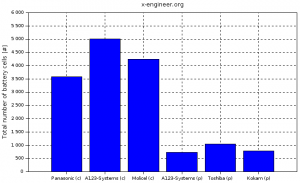Image: Battery pack total number of cells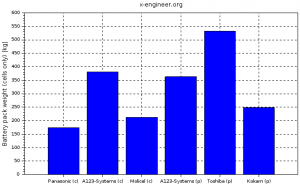Image: Battery pack mass (cells only)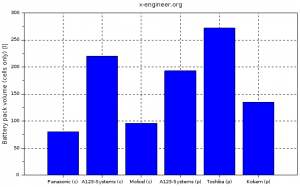Image: Battery pack volume (cells only)

Because of the low capacity for the cylindrical cells, compared to the pouch cells, the number of cells required for the battery pack is significant higher. A high number of cells might cause additional problems in areas of wiring, voltage monitoring, battery reliability.

The mass and volume are calculated only at cell level, taking into account the cell dimensions and mass. The battery pack which will be in the vehicle will have additional components (wires, electronic components, soldering, case, etc.), which will increase both the final volume and mass. Nevertheless by looking only at cell based volume and mass, we can estimate which model will be better compared to the other. In terms of mass and volume there is no clear distinction between cylindrical and pouch cells. However it seems that a battery pack with pouch cells is slightly heavier and bigger.

The battery cells manufactured by A123-Systems have very high maximum continuous discharge current and maximum pulse (peak) discharge current. As for energy and capacity, the pouch type cells have higher peak (continuous) current and power than cylindrical cells.

Based on the calculated data and conclusions, we can choose which battery cells are suitable for our electric vehicle battery pack. From our examples it looks like Kokam cells have the best compromise between mass, volume and energy/power density.

All the parameters, equations, results and plots are implemented in a Scilab (*.sce) file. For download please subscribe to the Patreon page.

You can also check your results using the calculator below.

### EV Battery Calculator (on-line)

 Vehicle range Average energy consumption Nominal battery voltage Dv [km] Eavg [Wh/km] Ubp [V] Cell type Lbc [m] Dbc [m] Hbc [m] Wbc [m] Tbc [m] CylindricalPouch mbc [kg] Cbc [Ah] Ubc [V] C-ratebcc [-] C-ratebcp [-] Battery Cell Performance Ebc [Wh] Vbc [l] uVbc [Wh/l] uGbc [Wh/kg] Battery Pack Performance # cells / string # strings # cells Es [Wh] Ebp [kWh] Cbp [Ah] mbp [kg] Vbp [l] Ibpp [A] Pbpp [kW] Ibpc [A] Pbpc [kW]

### References:

 Mooy, Robert & Aydemir, Muhammed & Seliger, Günther. (2017). Comparatively Assessing different Shapes of Lithium-ion Battery Cells. Procedia Manufacturing. 8. 104-111. 10.1016/j.promfg.2017.02.013.
 Bernardini, Annalia & Barrero, Ricardo & Macharis, Cathy & Van Mierlo, Joeri. (2015). Technological solutions aiming at recovering metro braking energy: a multi-criteria analysis case study. BDC – Bollettino del Centro Calza Bini – Università degli Studi di Napoli Federico II. 14. 301-325. 10.6092/2284-4732/2929.
 Tom Denton, Automobile Electrical and Electronic Systems, Third edition. Elsevier Butterworth-Heinemann, 2004, page 129.
 https://industrial.panasonic.com/
 http://www.a123systems.com/
 http://www.molicel.com/
 http://www.a123systems.com/
 http://www.toshiba.com/
 http://www.kokam.com/

#### Ad Blocker Detected

Dear user, Our website provides free and high quality content by displaying ads to our visitors. Please support us by disabling your Ad blocker for our site. Thank you!